Courses

# Test: Vector Algebra (Competition Level) - 3

## 25 Questions MCQ Test Mathematics (Maths) Class 12 | Test: Vector Algebra (Competition Level) - 3

Description
This mock test of Test: Vector Algebra (Competition Level) - 3 for JEE helps you for every JEE entrance exam. This contains 25 Multiple Choice Questions for JEE Test: Vector Algebra (Competition Level) - 3 (mcq) to study with solutions a complete question bank. The solved questions answers in this Test: Vector Algebra (Competition Level) - 3 quiz give you a good mix of easy questions and tough questions. JEE students definitely take this Test: Vector Algebra (Competition Level) - 3 exercise for a better result in the exam. You can find other Test: Vector Algebra (Competition Level) - 3 extra questions, long questions & short questions for JEE on EduRev as well by searching above.
QUESTION: 1

Solution:
QUESTION: 2

Solution:
QUESTION: 3

###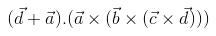simplifies to

Solution:
QUESTION: 4

Let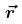be a vector perpendicular to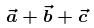, where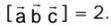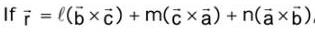then (ℓ +m+n) is equal to

Solution:
QUESTION: 5

If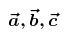are three non-coplaner non-zero vectors and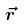is any vector in space, then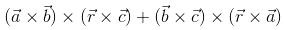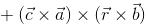is equal to

Solution:
QUESTION: 6

If a, b, c are pth, qth, rth terms of an H.P and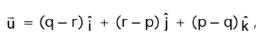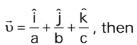Solution:
QUESTION: 7

If the unit vectors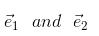are inclined at an angle 2θ and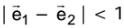, then for θ ∈ [0, π], θ may lie in the interval

Solution:
QUESTION: 8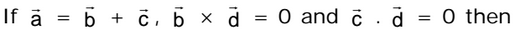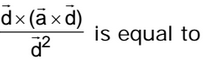Solution:
QUESTION: 9

Consider a tetrahedron with faces f1, f2, f3, fLet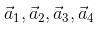​ be the vectors whose magnitudes are respectively equalt to the areas of  f1, f2, f3, f4 and  whose directions are perpendicular to these faces in the outward direction. Then

Solution:
QUESTION: 10

Let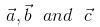be non-coplaner unit vectors equally inclined to one another at an acute angle θ. Then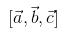in terms of  θ is equalt to

Solution:
QUESTION: 11

Let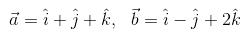and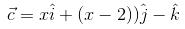.  If the vector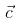lies in the plane of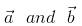, then x equals

Solution:
QUESTION: 12

Images of the point P with position vector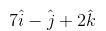in the line whose vector equation is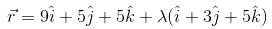has the position vector

Solution:
QUESTION: 13

Let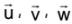be such that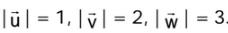. If the projection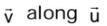is equal to that of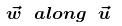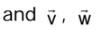are  perpendicular  to each other, then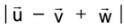equals.

Solution:
QUESTION: 14

Let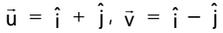and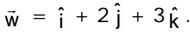. If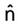is a unit vector such that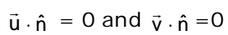then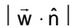is equal to

Solution:
QUESTION: 15

Equation of a line which passes through a point with position vector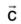, parallel to the plane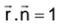and perpendicular to the line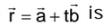Solution:
QUESTION: 16

Points L, M and N lie on the sides AB, BC and CA of the triangle ABC such that ℓ(AL) : ℓ(LB) = ℓ(BM) :ℓ(MC) = ℓ(CN) : ℓ(NA) = m :n, then the areas of the triangles LMN and ABC are in the ratio

Solution:
QUESTION: 17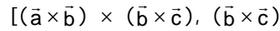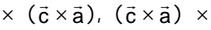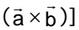is equal to

Solution:

Solution :- [a×b b×c c×a]

= (a×b) . [(b×c) * (c×a)]

= (a×b) [(b×c) . a(c) - (b×c) . c]

= (a×b) [(b×c) . a(c) - 0]

= [(a×b) . c] [(b×c) . a]

= [a b c] [(b c a]

= - [a b c] [(a c b]

= [a b c] [(a b c ]   => [a b c]^2

QUESTION: 18

If the vectors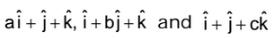(a ≠ b ≠ c ≠ 1) are coplanar, then the value of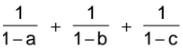is equal to

Solution:
QUESTION: 19

The vectors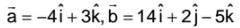are coinitial. The vector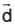which is bisecting the angle between the vectors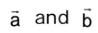and is having the magnitude √6 is

Solution:
QUESTION: 20

If A1, A2, A3, .........Aare the vertics of a regular plane polygon with n sides and O is its centre then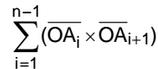equals

Solution:
QUESTION: 21

For any four points P,Q, R, S,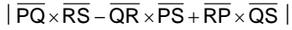is equal to 4 times the

area of the triangle

Solution:
*Multiple options can be correct
QUESTION: 22

If a line has a vector equation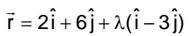, then which of the following statements hold goods ?

Solution:
*Multiple options can be correct
QUESTION: 23

The vector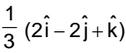is

Solution:
*Multiple options can be correct
QUESTION: 24

The vector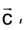directed along the external bisector of the angle between the vectors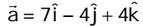and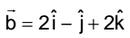with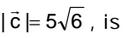Solution:
QUESTION: 25

Which of the following acts in accordance with right hand screw rule?

Solution: